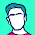## Saturday, April 24, 2010

### On the all other products, no division problem

This is among my favorite interview questions:
Given an array of numbers, return an array of the products of all other elements in the array.

More formally, write a method `int[] products(int...)`, where `products(nums)[i]` is the product of all `nums[j], j != i`.
```System.out.println(
java.util.Arrays.toString(
products(1, 2, 3, 4, 5)
)
); // prints "[120, 60, 40, 30, 24]"
// i.e. [(2*3*4*5), (1*3*4*5), (1*2*4*5), (1*2*3*5), (1*2*3*4)]
```
You must do this in `O(N)` without using division.
I offer two solutions, both beautiful and hideous in their own ways.

## Iterative

```static int[] products(int... nums) {
final int N = nums.length;
int[] prods = new int[N];
java.util.Arrays.fill(prods, 1);
for (int // pi----> * <----pj
i = 0, pi = 1    ,  j = N-1, pj = 1  ;
(i < N)           &          (j >= 0) ;
pi *= nums[i++]  ,  pj *= nums[j--]  )
{
prods[i] *= pi   ;  prods[j] *= pj   ;
}
return prods;
}
```
`O(N)` space for output, `O(1)` temporary, `O(N)` time, no division. The formatting betrays all conventions, but self-documentation doesn't get any more poetic than this.

## Recursive

```static int[] products(int... nums) {
products(nums, 1, 0);
return nums;
}
static int products(int[] nums, int p, int n) {
return (n == nums.length) ? 1
: nums[n] * (p = products(nums, nums[n] * (nums[n] = p), n + 1))
+ 0*(nums[n] *= p);
}
```
In-place modification of the input array, `O(N)` temporary space on the stack, `O(N)` time, no division. The less said about it the better.

1.2.